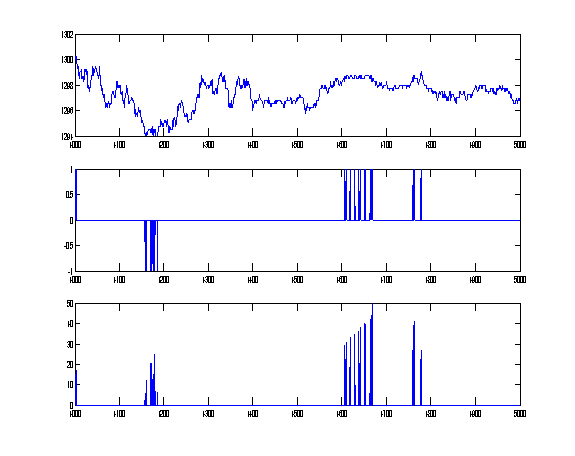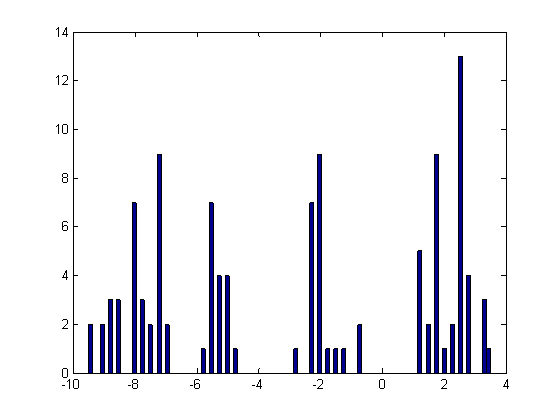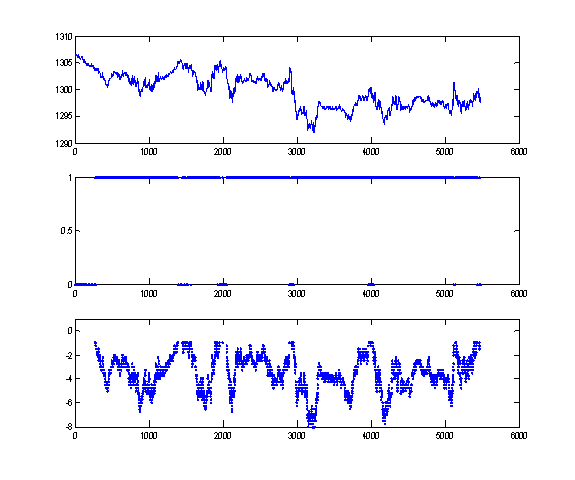Daily Speculations The Web Site of Victor Niederhoffer and Laurel KennerDaily Speculations

# 1         Intro

It was proposed to examine a timeseries of data for the purpose of counting intraday turnarounds, subsequent price breakouts, and to compare this behavior to non-turnaround behavior.Figure 0. Exemplar data series motivating analysis

# 2         Data Source

Figure 1 is a plot of tick ESU6 timeseries data.  There is so much “jitter” in this signal, that it is a challenge to decide on a general definition for a turning point for counting purposes.  There can be hundreds of local extrema in a target range over the course of reasonable time windows.  Using 1-minute data provides a much smoother data set; however, this begs the question of what information has been lost.  The subsequent analysis was performed using the reported closing price on one-minute data bars for the week of 8/21 – 8/25 of the ESU6 contract as shown in Figure 2.  Special attention was focused on the period 8/24 – 8/25 as shown in Figure 3 since there is a visible resistance level at 1299 that is tentatively broken once, then re-established, then finally broken by the end of trading on the 25th.Figure  SEQ Figure \* ARABIC 1. ESU6 Timeseries Tick DataFigure 2. ESU6 One Minute Data 8/21/06 - 8/25/06Figure 3. ESU6 One Minute Data 8/24/06 - 8/25/06

# 3         Turnarounds

Figure 4 shows a segment of ESU6 data focusing on a series of turnarounds near the 1299 level following the brief breakout above this level at 1300 on the 24th.  The analysis uses a +/- 0.5 price band for turning point detection and requires that a turning point be the highest or lowest price in the last 0.5 days.  The window for the counting of successive extrema at a given level (within the given range) is 0.5 days.  Figure 5 shows similar data for the entire week of data.Figure 4. ESU6 Turning Point Counts near 1299, price tolerance +/- 0.5, counting window 0.5 days, extrema significance window 0.5 days (A: Time series; B: 1 = Maxima, -1 = Minima; C: Counting of Exterma)Figure 5. ESU6 8/2/06 – 8/25/06 Turning Point Counts, price tolerance +/- 0.5, counting window 0.5 days, extrema significance window 0.5 days (A: Time series; B: 1 = Maxima, -1 = Minima; C: Counting of Exterma)

# 4         Breakouts

Figure 6 shows the focus section of data for which the identified turning points are subsequently broken out either high for a maxima or low for a minima.  The breakout search window is 1 day.  Breakouts were identified as the maximum (for maxima turning points) and minimum (for minima turning points) level attained by the price in the search window during the breakout activity.  The graph also shows the price level of the breakout over the turning point level.  The level of this breakout is around 3 points and occurs later in the day on Friday.  Figure 7 shows similar charts for the week.  Breakouts were usually less than 5 points, but a couple downward events had a magnitude of nearly 10.Figure 6. ESU6 Breakouts focused on 1299 turning point region, breakout search forward 1 day (A: Time series; B: 1 = Maxima Breakout, -1 = Minima Breakout; C: Price Level of Breakout)Figure 7. ESU6 8/21/06 – 8/25/06 breakout search forward 1 day (A: Time series; B: 1 = Maxima Breakout, -1 = Minima Breakout; C: Price Level of Breakout)

# 5         Statistics

Figure 8 shows that not all identified turning points are followed by a price breakout.  In fact, 32.84% of them were.  The mean breakout size was 4.3125 and the standard deviation was 2.671.  Figure 9 shows a histogram of Breakout Level past Turning Points.Figure 8. ESU6 8/21/06 – 8/25/06 Breakouts vs. Turning Points (A: Time series; B: 1 = Maxima, -1 = Minima; C: Price Level of Breakout)Figure 9. Histogram of Breakout Level past identified turning points in ESU6 8/21 – 8/25

The minimum breakout level of the detected turnarounds is 0.75.  This is the level used in the subsequent analysis of the general price timeseries.

## 5.1      Non-Turnaround Analysis

Price movements greater than 0.75 were detected for each datapoint in the series within a 0.5 day time window.  Since a standard metric for whether a given data point was part of an up trend or a down trend was not used, each data point was analyzed for price movements greater than and less than the threshold level.

Figure 10 shows the analysis for “breakouts’ above the current price.  Figure 11 shows the analysis for “breakouts” below the current price.  The high breakout rate was 88.8 %.  The low breakout rate was 93.9%.Figure 10. “Breakouts” High not associated with turnarounds, 1 day search window, 0.75 threshold (A: Time series; B: 1 = Breakout, 0 = no breakout; C: Breakout level)Figure 11. “Breakouts” Low not associated with turnarounds, 1 day search window, 0.75 threshold (A: Time series; B: 1 = Breakout, 0 = no breakout; C: Breakout level)

## 5.2      Possible Effect of Turning Points

Figure 12 compares identified Turning Points to non-turning point “breakouts.”  Subchart C shows that some of the identified turning points are associated with the limited set of data that did not “breakout.”  16 % of the identified turning points were so associated.  49% of the subset of turning points that did not breakout corresponded with such points.  Naturally, these turning points were those that themselves did not subsequently breakout, as shown in subchart E.  One possible conclusion is that an identified turning point limits the probability that the price will move outside of a range during a given time window.Figure 12. Turning Point vs. Non-Turning Point Breakouts.  (A: Time Series; B: Non-turning point breakouts, 0 = no breakout; C: Turning points; D: Turning points that match no non-turning point breakout; E: Points in “d” that breakout (null))

# 6         Next Steps

The various processing parameters (turning point counting time window, breakout search time window, etc.) should be used as parameters on a multi-run analysis on a much larger data set to find a definition of a turning point to use in practice.  It is possible that this definition may change with prevailing conditions, in which case dynamic windows could be implemented.  A proposed deciding metric could be a discrimination function between the probability of the turning point bounding price behavior and not.  Various binary classification schemes are available for testing under this scheme.